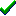# fg_3Dsetobject()Win32DirectXFastgraph/Light

Prototype

void fg_3Dsetobject (double x, double y, double z, int x_angle, int y_angle, int z_angle);

Sub fg_3Dsetobject (ByVal x As Double, ByVal y As Double, ByVal z As Double, ByVal x_angle As Long, ByVal y_angle As Long, ByVal z_angle As Long)

procedure fg_3Dsetobject (x, y, z : double; x_angle, y_angle, z_angle : integer);

Description

The fg_3Dsetobject() function defines an object's position in 3D world space and its rotation about its own object space axes (center of gravity).

Parameters

x is the x position of the object's center of gravity in 3D world space.

y is the y position of the object's center of gravity in 3D world space.

z is the z position of the object's center of gravity in 3D world space.

x_angle is the counterclockwise rotation about the object's x axis, expressed in tenths of degrees.

y_angle is the counterclockwise rotation about the object's y axis, expressed in tenths of degrees.

z_angle is the counterclockwise rotation about the object's z axis, expressed in tenths of degrees.

Return value

none

Restrictions

none

See also

Examples

Contents
Fastgraph Home Page

copyright 2001 Ted Gruber Software, Inc.